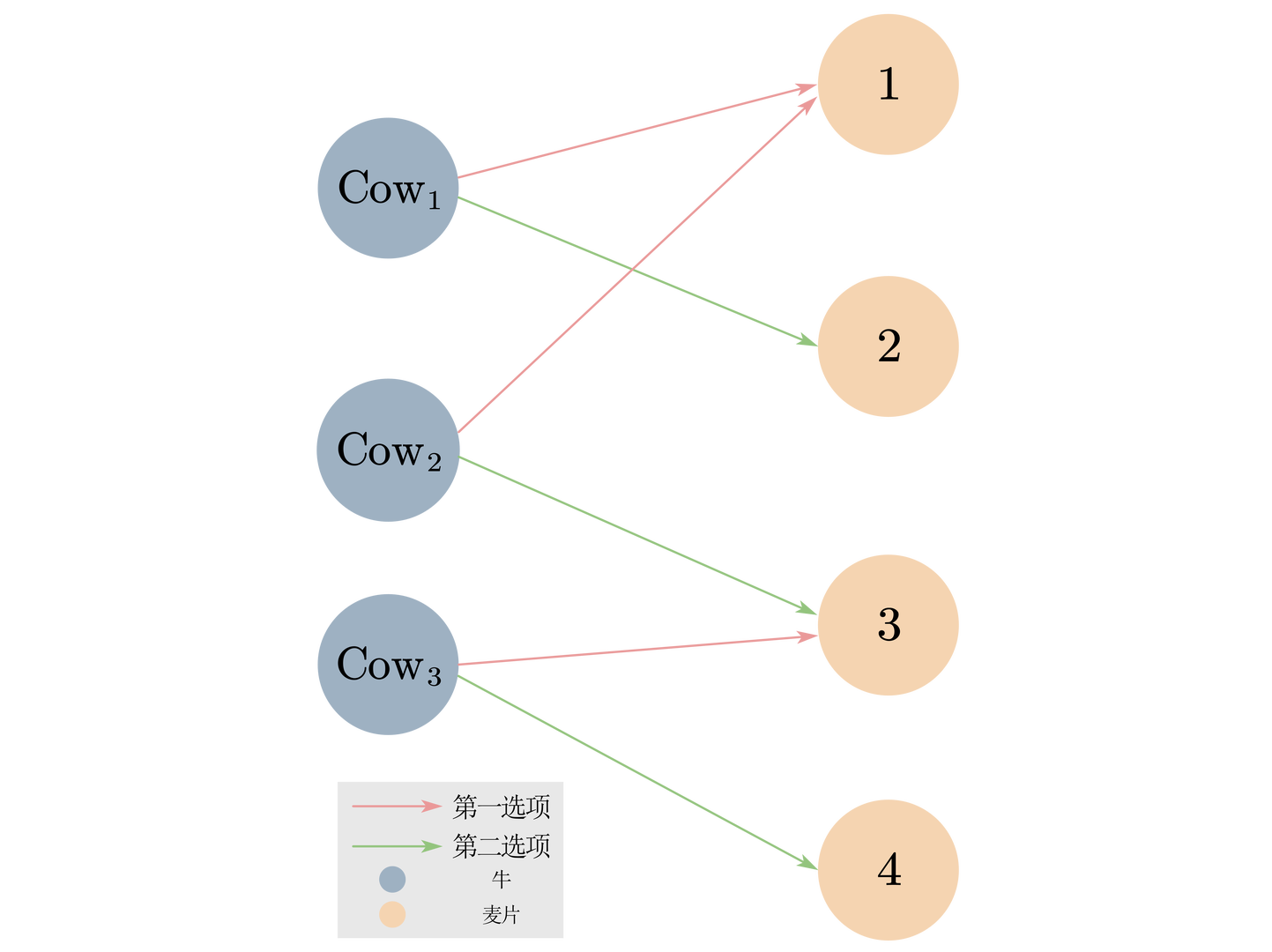update@2022/3/13: 感谢@小木虫的提醒，当前的解法不是正解！ 如果USACO的数据够强的话，目前我使用的匈牙利算法因为复杂度是 $O(nm)$，是过不了这道题的。如果想用我这个二分图匹配 + 拓扑的方法实现，可以使用dinic算法求二分图最大匹配（不过写起来会比较麻烦）。本人有时间的时候也会尝试用dinic实现这个解法并且更新题解。

# 1：题意

$N$ 头牛，$M$ 种麦片（每种一箱），每头都有第一和第二喜欢的麦片种类（下文简称为一选和二选），牛会优先选择自己最喜欢的麦片，当最喜欢的麦片被占用后会选择第二喜欢的麦片，问：

1. 最少会有多少牛得不到麦片。
2. 能达到此最小值的牛的排列

# 2：分析

## 2.2 第二小问

1 (cow) -> [1 (fir), 2 (sec)]
2 (cow) -> [1 (fir), 3 (sec)]
3 (cow) -> [3 (fir), 4 (sec)]1. $\text{Cow}_1$ 会先选择它的一选，也就是麦片 1
2. 因为 $\text{Cow}_2$ 的一选被 $\text{Cow}_1$ 占用了，所以它会选择它的二选，也就是麦片 3
3. $\text{Cow}_3$ 的一选被 $\text{Cow}_2$ 占用了，所以它会选择他的二选，也就是麦片 4

1. $\text{Cow}_1$ 会选择它的一选，也就是麦片 1
2. $\text{Cow}_3$ 会选择它的一选，也就是麦片 3
3. $\text{Cow}_2$ 的一选和二选都被占用了（麦片 1 3），它不能吃到麦片

# 3：算法过程

for(int i = 1; i <= n; i++){              //i遍历的是成功匹配到一选的奶牛
if(invmatched[i] != e[i]) continue;//e[i]表示i的一选
//invmatched[i] 是i最终匹配到的麦片
//所以这句话的意思是如果不是一选就直接continue
printf("%d\n",i);//如果是一选直接输出

for(int cur:inve[e[i]]){         //遍历当前成功匹配一选的牛 可能影响的牛
//e[i]表示的是i的一选，而i一定是成功匹配一选的牛
//inve[e[i]]是所有一选和i的一选相同的奶牛，这些奶牛可能被i影响
if(invmatched[cur] == e[cur])//前文提到了invmatched[cur] 表示的是cur最终匹配到的麦片
//而e[cur] 表示的是 cur 的二选
{                               //所以这句话确保了cur最终选到的是二选（说明这头牛被影响到了，没有选一选，同时也可以防止把i自己入队）
q.push(cur);
}
}
}


while(!q.empty()){
int cur = q.front();
printf("%d\n",cur);//队列是先进先出的结构，所以可以先输出在影响链上方的牛（更早被影响到的牛）
q.pop();
for(int nex:inve[e[cur]]){         //e[cur]是编号为cur的牛的二选
//inve[e[cur]] 就是所有把 cur 的二选当作一选的牛，也就是可能被 cur 影响到的牛
if(invmatched[nex] == e[nex]) {//最终选到的是二选（说明这头牛被影响到了）
q.push(nex);
}
}
}

# 4：代码实现以及细节

/*Date: 22 - 02-03 22 19
PROBLEM_NUM: P8095 [USACO22JAN] Cereal 2 S*/
#include<bits/stdc++.h>
using namespace std;
const int MAXN = 2e5 + 10;
int n, m;
vector<int> e[MAXN], inve[MAXN];
queue<int> q;
int vised[MAXN], matched[MAXN];
int invmatched[MAXN];

bool found(int cur){//匈牙利算法
for(int nex:e[cur]){
if(vised[nex]) continue;
vised[nex] = true;
if(!matched[nex] || found(matched[nex])){
matched[nex] = cur;
invmatched[cur] = nex;
vised[nex] = false;
return true;
}
}
return false;
}

int main(){
int match_cnt = 0;
scanf("%d%d",&n,&m);
for(int i = 1; i<=n; i++){
int f,s;
scanf("%d%d",&f,&s);
e[i].push_back(f);   //e[i]是i的一选
e[i].push_back(s);   //e[i]是i的二选
inve[f].push_back(i);//inve[f] 表示所有把 f 号麦片作为一选的牛
}
for(int i = 1;i <= n; i++){//匈牙利算法部分
if(found(i)){
match_cnt++;
}
}

printf("%d\n", n - match_cnt);//饥饿的奶牛 = 所有奶牛 - 吃到麦片的奶牛

for(int i = 1; i <= n; i++){          //i遍历的是成功匹配到一选的奶牛
if(invmatched[i] != e[i]) continue;//如果不是一选就直接continue
printf("%d\n",i);                     //如果是一选直接输出

for(int cur:inve[e[i]]){         //遍历当前成功匹配一选的牛 可能影响的牛
//e[i]表示的是i的一选，而i一定是成功匹配一选的牛
//inve[e[i]]是所有一选和i的一选相同的奶牛，这些奶牛可能被i影响
if(invmatched[cur] == e[cur])//前文提到了invmatched[cur] 表示的是cur最终匹配到的麦片
//而e[cur] 表示的是 cur 的二选
{                           //所以这句话确保了cur最终选到的是二选（说明这头牛被影响到了，没有选一选，同时也可以防止把i自己入队）
q.push(cur);
}
}
}

while(!q.empty()){
int cur = q.front();
printf("%d\n",cur);//队列是先进先出的结构，所以可以先输出在影响链上方的牛（更早被影响到的牛）
q.pop();
for(int nex:inve[e[cur]]){         //e[cur]是编号为cur的牛的二选
//inve[e[cur]] 就是所有把 cur 的二选当作一选的牛，也就是可能被 cur 影响到的牛
if(invmatched[nex] == e[nex]) {//最终选到的是二选（说明这头牛被影响到了）
q.push(nex);
}
}
}

for(int i = 1; i<=n; i++){//最后输出没有成功匹配到的奶牛
if(!invmatched[i]){   //invmatched[i] == 0 说明奶牛 i 没有匹配到任何麦片
printf("%d\n",i);
}
}

system("pause");
}